﻿ Optimization in Deteriorated Inventory Model Incorporated with Percentage Loss, Promotion and Functional Ordering Cost: A Comparative Analysis

### Optimization in Deteriorated Inventory Model Incorporated with Percentage Loss, Promotion and Functi...

Monalisha Pattnaik, Purnima Nayak, Chandni AgarawalOPEN ACCESSPEER-REVIEWED

## Optimization in Deteriorated Inventory Model Incorporated with Percentage Loss, Promotion and Functional Ordering Cost: A Comparative Analysis

Monalisha Pattnaik1,, Purnima Nayak2, Chandni Agarawal1

2Department Mathematics, P.N. (Auto) College, Khorda, Bhubaneswar

### Abstract

This paper explores the instantaneous economic order quantity model by allocating the percentage of units lost due to deterioration in an on-hand inventory by framing promotional effort cost and variable ordering cost. The objective is to maximize the net profit so as to determine the order quantity, promotional effort factor, the cycle length and number of units lost due to deterioration. For any given number of replenishment cycles the existence of a unique optimal replenishment schedule are proved and mathematical model is developed to find some important characteristics for the concavity of the net profit function. Numerical examples are provided to illustrate the results of proposed model which benefit the retailer and this policy is important, especially for wasting of deteriorating items. Finally, sensitivity analyses of the optimal solution with respect to the major parameters and comparative analysis of different related EOQ models are also carried out.

### At a glance: Figures

1
Prev Next

• Pattnaik, Monalisha, Purnima Nayak, and Chandni Agarawal. "Optimization in Deteriorated Inventory Model Incorporated with Percentage Loss, Promotion and Functional Ordering Cost: A Comparative Analysis." Journal of Business and Management Sciences 2.3A (2014): 21-28.
• Pattnaik, M. , Nayak, P. , & Agarawal, C. (2014). Optimization in Deteriorated Inventory Model Incorporated with Percentage Loss, Promotion and Functional Ordering Cost: A Comparative Analysis. Journal of Business and Management Sciences, 2(3A), 21-28.
• Pattnaik, Monalisha, Purnima Nayak, and Chandni Agarawal. "Optimization in Deteriorated Inventory Model Incorporated with Percentage Loss, Promotion and Functional Ordering Cost: A Comparative Analysis." Journal of Business and Management Sciences 2, no. 3A (2014): 21-28.

 Import into BibTeX Import into EndNote Import into RefMan Import into RefWorks

### 1. Introduction

Most of the literature on inventory control and production planning has dealt with the assumption that the demand for a product will continue infinitely in the future either in a deterministic or in a stochastic fashion. This assumption does not always hold true. Inventory management plays a crucial role in businesses since it can help companies reach the goal of ensuring prompt delivery, avoiding shortages, helping sales at competitive prices and so forth. The mathematical modeling of real-world inventory problems necessitates the simplification of assumptions to make the mathematics flexible. However, excessive simplification of assumptions results in mathematical models that do not represent the inventory situation to be analyzed.

Many models have been proposed to deal with a variety of inventory problems. The classical analysis of inventory control considers three costs for holding inventories. These costs are the procurement cost, carrying cost and shortage cost. The classical analysis builds a model of an inventory system and calculates the EOQ which minimize these three costs so that their sum is satisfying minimization criterion. One of the unrealistic assumptions is that items stocked preserve their physical characteristics during their stay in inventory. Items in stock are subject to many possible risks, e.g. damage, spoilage, dryness; vaporization etc., those results decrease of usefulness of the original one and a cost is incurred to account for such risks.

The EOQ inventory control model was introduced in the earliest decades of this century and is still widely accepted by many industries today. Comprehensive reviews of inventory models can be found in Osteryoung, Mccarty and Reinhart (1986)  and Pattnaik (2013) . In previous deterministic inventory models, many are developed under the assumption that demand is either constant or stock dependent for deteriorated items. Jain and Silver (1994)  developed a stochastic dynamic programming model presented for determining the optimal ordering policy for a perishable or potentially obsolete product so as to satisfy known time-varying demand over a specified planning horizon. They assumed a random lifetime perishability, where, at the end of each discrete period, the total remaining inventory either becomes worthless or remains usable for at least the next period. Mishra (2012)  explored the inventory model for time dependent holding cost and deterioration with salvage value where shortages are allowed. Gupta and Gerchak (1995)  examined the simultaneous selection product durability and order quantity for items that deteriorate over time. Their choice of product durability is modeled as the values of a single design parameter that effects the distribution of the time-to-onset of deterioration (TOD) and analyzed two scenarios; the first considers TOD as a constant and the store manager may choose an appropriate value, while the second assumes that TOD is a random variable. Goyal and Gunasekaran (1995)  considered the effect of different marketing policies, e.g. the price per unit product and the advertisement frequency on the demand of a perishable item. Bose, Goswami and Chaudhuri (1995)  considered an economic order quantity (EOQ) inventory model for deteriorating goods developed with a linear, positive trend in demand allowing inventory shortages and backlogging. Bose, Goswami and Chaudhuri (1995)  and Hariga (1996)  investigated the effects of inflation and the time-value of money with the assumption of two inflation rates rather than one, i.e. the internal (company) inflation rate and the external (general economy) inflation rate. Hariga (1994)  argued that the analysis of Bose, Goswami and Chaudhuri (1995)  contained mathematical errors for which he proposed the correct theory for the problem supplied with numerical examples. Padmanabhan and Vrat (1995)  presented an EOQ inventory model for perishable items with a stock dependent selling rate. They assumed that the selling rate is a function of the current inventory level and the rate of deterioration is taken to be constant. Pattnaik (2012)  explained a non-linear profit-maximization entropic order quantity model for deteriorating items with stock dependent demand rate. Pattnaik (2013)  introduced a fuzzy EOQ model with demand dependent unit cost and varied setup cost under limited storage capacity.

The most recent work found in the literature is that of Hariga (1995)  who extended his earlier work by assuming a time-varying demand over a finite planning horizon. Salameh, Jabar and Nouehed (1999)  studied an EOQ inventory model in which it assumes that the percentage of on-hand inventory wasted due to deterioration is a key feature of the inventory conditions which govern the item stocked.

Pattnaik (2012)  introduced an EOQ model for perishable items with constant demand and instant deterioration. Pattnaik (2012)  studied the effect of promotion in fuzzy optimal replenishment model with units lost due to deterioration. Pattnaik (2013)  investigated linear programming problems in fuzzy environment with evaluating the post optimal analyses. Raafat (1991)  explained survey of literature on continuously deteriorating inventory models. Roy and Maiti (1997)  presented fuzzy EOQ model with demand dependent unit cost under limited storage capacity. Tripathy, Pattnaik and Tripathy (2012)  introduced optimal EOQ model for Deteriorating Items with Promotional Effort Cost. Tripathy, Pattnaik and Tripathy (2013)  presented a decision-making framework for a single item EOQ model with two constraints. Tsao and Sheen (2008)  explored dynamic pricing, promotion and replenishment policies for a deteriorating item under permissible delay in payment. Waters (1994)  and Pattnaik (2012)  and Pattnaik (2014)  defined various inventory models with managerial decisions. Wee (1993)  explained an economic production lot size model for deteriorating items with partial back-ordering. In this paper, replenishment decision under wasting the percentage of on-hand inventory due to deterioration are adjusted arbitrarily upward or downward for profit maximization model in response to the change in market demand within the finite planning horizon with dynamic setup cost with promotional effort cost. The objective of this paper is to determine optimal replenishment quantities in an instantaneous replenishment profit maximization model.

All mentioned above inventory literatures with deterioration or no wasting the percentage of on-hand inventory due to deterioration have the basic assumption that the retailer owns a storage room with optimal order quantity. In recent years, companies have started to recognize that a tradeoff exists between product varieties in terms of quality of the product for running in the market smoothly. In the absence of a proper quantitative model to measure the effect of product quality of the product, these companies have mainly relied on qualitative judgment. This paper postulates that measuring the behavior of production systems may be achievable by incorporating the idea of retailer in making optimum decision on replenishment with wasting the percentage of on-hand inventory due to deterioration with dynamic ordering cost and then compares the optimal results with no wasting the percentage of on-hand inventory with promotional effort cost due to deterioration traditional model. The major assumptions used in the above research articles are summarized in Table 1.

#### Table 1. Summary of the Related Researches

The remainder of the paper is organized as follows. In section 2 assumptions and notations are provided for the development of the model. The mathematical formulation is developed in section 3. The solution procedure is given in section 4. In section 5, numerical example is presented to illustrate the development of the model. The sensitivity analysis is carried out in section 6 to observe the changes in the optimal solution. The comparative analysis of different related EOQ models with the present models is carried out in section 7 to study effectiveness of the current model. Finally section 8 deals with the summary and the concluding remarks.

### 2. Notations and Assumptions

r: Consumption rate

tc: Cycle length

h: Holding cost of one unit for one unit of time.

HC (q): Holding cost per cycle

Ps: Selling Price per unit

α: Percentage of on-hand inventory that is lost due to deterioration

q: Order quantity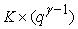: Ordering cost per cycle (OC) where, 0<γ<1

q**: Modified economic ordering / production quantity (EOQ/EPQ)

q*: Traditional economic ordering quantity (EOQ)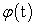: On-hand inventory level at time t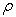: The promotional effort factor per cycle.

PE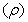: The promotional effort cost, PE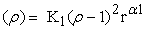where,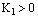and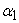is a constant.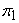(q, ρ): Net profit per unit of producing q units per cycle in crisp strategy.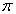(q, ρ): Average profit per unit of producing q units per cycle in crisp strategy.

### 3. Mathematical Model

Denote φ(t) as the on-hand inventory level at time t. During a change in time from point t to t+dt, where t + dt > t, the on-hand inventory drops from φ(t) to φ(t+dt). Then φ(t+dt) is given as: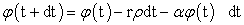(1)

Equation (1) can be re-written as: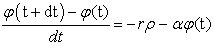(2)

and dt → 0, equation (2) reduces to: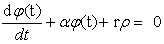(3)

Equation (3) is a differential equation, solution is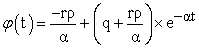(4)

Where q is the order quantity which is instantaneously replenished at the beginning of each cycle of length tc units of time. The stock is replenished by q units each time these units are totally depleted as a result of outside demand and deterioration. The cycle length, tc, is determined by first substituting tc into equation (4) and then setting it equal to zero to get: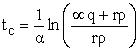(5)

Equation (4) and (5) are used to develop the mathematical model. It is worthy to mention that as α approaches to zero, tc approaches to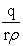. Then the total number of units lost per cycle, L, is given as: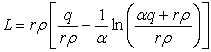(6)

The total cost per cycle, TC(q), is the sum of the variable ordering cost and purchasing cost per cycle,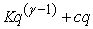, the holding cost per cycle, HC(q), and the promotional effort cost per cycle, PE(ρ). HC (q) is obtained from equation (4) as :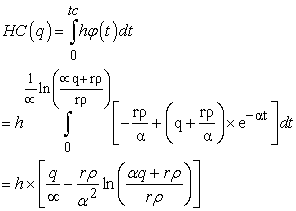(7)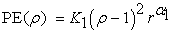(8)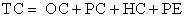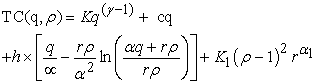(9)

The total cost per unit of time, TCU (q,ρ), is given by dividing equation (9) by equation (5) to give: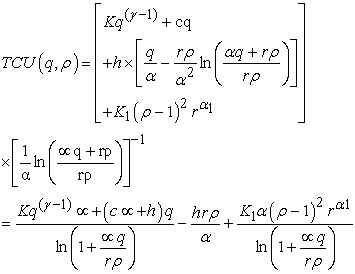(10)

As α approaches zero and ρ = 1 equation (10) reduces to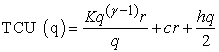Whose solution is given by the traditional EOQ formula,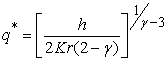. The total profit per cycle is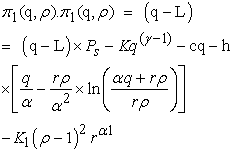(11)

Where L, the number of units lost per cycle due to deterioration, and TC (q, ρ) the total cost per cycle, are calculated from equations (6) and (9), respectively. The average profit π(q, ρ) per unit time is obtained by dividing tc in π1(q, ρ). Hence the profit maximization problem is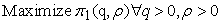(12)

### 4. Solution Procedure (Optimization)

The optimal ordering quantity q and promotional effort ρ per cycle can be determined by differentiating equation (12) with respect to q and ρ separately, setting these to zero.

In order to show the uniqueness of the solution in, it is sufficient to show that the net profit function throughout the cycle is jointly concave in terms of ordering quantity q and promotional effort ρ. The second partial derivates of equation (12) with respect to q and ρ are strictly negative and the determinant of Hessian matrix is positive. We consider the following propositions.

Proposition 1 The net profit(q, ρ) per cycle is concave in q.

Conditions for optimal q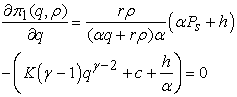(13)

The second order partial derivative of the net profit per cycle with respect to q can be expressed as: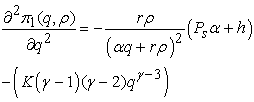(14)

Since rρ> 0,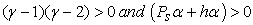Equation (14) is negative.

Proposition 2 The net profit(q, ρ) per cycle is concave in ρ.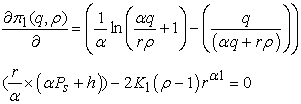(15)

The second order partial derivative of the net profit per cycle with respect to ρ is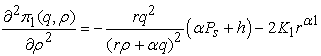(16)

Since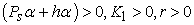, it is found that Eq. (16) is negative.

Propositions 1 and 2 show that the second partial derivatives of equation (12) with respect to q and ρ separately are strictly negative. The next step is to check that the determinant of the Hessian matrix is positive, i.e.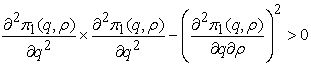(17)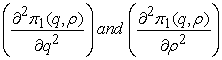shown in Equations (13 & 15) and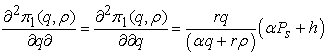(18)

The net profit per unit time we have the following maximization problem.

Maximize(q, ρ)

Subject to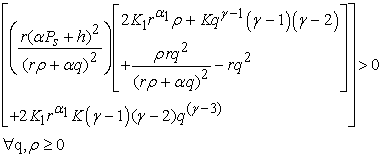(19)

The objective is to determine the optimal values of q and ρ to maximize the net profit function. It is very difficult to derive the optimal values of q and ρ, hence unit profit function. There are several methods to cope with constraints optimization problem numerically. But here we use LINGO 13.0 software to derive the optimal values of the decision variables.

### 5. Numerical Example

Consider an inventory situation where K is Rs. 200 per order, h is Rs. 5 per unit per unit of time, r is 1200 units per unit of time, c is Rs. 100 per unit, the selling price per unit Ps is Rs. 125, γ is 0.5 and α is 5%, K1=200 and α1=1.0. The optimal solution that maximizes equation (12) and q** and ρ* are determined by using LINGO 13.0 version software and the results are tabulated in Table 2. In the present model the net profit, units lost due to deterioration, the cycle length and order quantity are comparatively more than that of the comparative models, it indicates the present model incorporated with promotional effort cost, variable ordering cost and units lost due to deterioration may draw the better decisions in managerial uncertain space.

### 6. Sensitivity Analysis

It is interesting to investigate the influence of α on retailer behavior. The computational results shown in Table 3 indicates the following managerial phenomena: when the percentage of on hand inventory is lost due to deterioration i. e. α increases, the replenishment cycle length decreases, but the optimal replenishment quantity, the optimal total number of units lost per cycle and the optimal promotional effort, the optimal promotional effort cost and optimal total profit per unit of unit time and the optimal average profit per unit of unit time are decreasing and then increasing respectively. The optimal variable ordering cost increases then decreases with increase in α. Figure 1 represents the relationship between the order quantity q and dynamic setup cost OC. Figure 2 shows the relationship between the order quantity q and units lost per cycle due to deterioration L.

It is interesting to investigate the influence of the major parameters K, h, r, c, Ps, γ, K1 and α1 on retailer’s behaviour. The computational results shown in Table 4 indicate the following managerial phenomena:

tc the replenishment cycle length, q the optimal replenishment quantity, ρ the optimal promotional effort factor, L the optimal units lost due to deterioration, PE promotional effort cost, π1 the optimal net profit per unit per cycle and π the optimal average profit per unit per cycle are insensitive to the parameter K but OC variable setup cost is moderately sensitive to the parameter K.

tc the replenishment cycle length, q the optimal replenishment quantity, ρ the optimal promotional effort factor, L the optimal units lost due to deterioration, PE promotional effort cost, π1 the optimal net profit per unit per cycle and π the optimal average profit per unit per cycle are sensitive to the parameter h but OC variable setup cost is moderately sensitive to the parameter h.

tc the replenishment cycle length and ρ the optimal promotional effort factor are insensitive to the parameter r but q the optimal replenishment quantity, L the optimal units lost due to deterioration, PE promotional effort cost, π1 the optimal net profit per unit per cycle and π the optimal average profit per unit per cycle are sensitive to the parameter r and OC variable setup cost is moderately sensitive to the parameter r.

tc the replenishment cycle length, q the optimal replenishment quantity, ρ the optimal promotional effort factor, L the optimal units lost due to deterioration, PE promotional effort cost, OC variable setup cost, π1 the optimal net profit per unit per cycle and π the optimal average profit per unit per cycle are sensitive to the parameter c.

tc the replenishment cycle length, q the optimal replenishment quantity, ρ the optimal promotional effort factor, L the optimal units lost due to deterioration, PE promotional effort cost, OC variable setup cost, π1 the optimal net profit per unit per cycle and π the optimal average profit per unit per cycle are sensitive to the parameter Ps.

tc the replenishment cycle length and ρ the optimal promotional effort factor, q the optimal replenishment quantity, L the optimal units lost due to deterioration, PE promotional effort cost, π1 the optimal net profit per unit per cycle and π the optimal average profit per unit per cycle are insensitive to the parameter γ and OC variable setup cost is highly sensitive to the parameter γ.

tc the replenishment cycle length is insensitive to the parameter K1 but ρ the optimal promotional effort factor, q the optimal replenishment quantity, L the optimal units lost due to deterioration, OC variable setup cost, PE promotional effort cost, π1 the optimal net profit per unit per cycle and π the optimal average profit per unit per cycle are sensitive to the parameter K1.

tc the replenishment cycle length is insensitive to the parameter α1 but ρ the optimal promotional effort factor, q the optimal replenishment quantity, L the optimal units lost due to deterioration, OC variable setup cost, PE promotional effort cost, π1 the optimal net profit per unit per cycle and π the optimal average profit per unit per cycle are sensitive with static to the parameter α1.

#### Table 4. Sensitivity Analyses of the parameters K, h, r, c, Ps, γ, K1and α1

Figure 1. Two dimensional plot of Order Quantity, q and Dynamic Ordering Cost, OC.

### 7. Comparative Analysis

From Table 5, it can be found that the retailer always includes the wastage to percentage of on-hand inventory due to deterioration in EOQ model for obtaining the maximum net profit with less time consumption. Figure 3 represents the comparative study of order quantity and net profit of different related EOQ models. From Table 6, Table 7 and Table 8 it concludes that for all cases q** < q* and π1* < π1, which indicates that the present model is an effective one in comparison to other related EOQ models.

#### Table 5. Comparative Analysis of Different EOQ Models

Figure 3. Comparative Analysis of Order Quantity and Net Profit of Different EOQ Models

### 8. Conclusion

Now a days research on sales promotions has shed much light on the effects of price promotions. Promotional effort factor plays a significant role in framing the promotional effort cost. In this paper, it is analyzed that the effect of promotional effort cost for a modified EOQ model with a percentage of the on-hand inventory lost due to deterioration and variable ordering cost as characteristic features and the inventory conditions govern the item stocked. This paper provides a useful property for finding the optimal profit and ordering quantity with deteriorated units of lost sales. A new mathematical model with variable setup cost is developed and compared to the traditional EOQ model numerically. The economic order quantity, q** and the net profit for the modified model and the modified average profit per unit per cycle were found to be more than that of the traditional, q*, i.e. q**>q*, the net profit and average profit per unit per cycle respectively. Finally, wasting the percentage of on-hand inventory due to deterioration effect was demonstrated numerically to have an adverse effect on the average profit per unit per cycle. Hence the utilization of units lost due to deterioration and promotional effort cost makes the scope of the applications broader. Further, a numerical example is presented to illustrate the theoretical results, and some observations are obtained from sensitivity analysis with respect to the major parameters α, K, h, r, c, Ps, γ, K1 and α1. The model in this study is a general framework that considers wasting/ no wasting the percentage of on-hand inventory due to deterioration with promotional effort cost and variable ordering cost simultaneously. To the best of its knowledge, this is the article that investigates the impact of promotions, units lost due to deterioration and variable ordering cost simultaneously on crisp-type environment.

There are many scopes in extending the present work as a future research work. Parameters and decision variables can be considered random or even fuzzy. Effect of shortage, backlogging inflation etc could be added to the multi-item model.

### References

  Bose, S., Goswami, A. and Chaudhuri, K.S. (1995). An EOQ model for deteriorating items with linear time-dependent demand rate and shortages under inflation and time discounting. Journal of Operational Research Society, 46: 775-782.In article CrossRef  Goyal, S.K. and Gunasekaran, A. (1995). An integrated production-inventory-marketing model for deteriorating items. Computers and Industrial Engineering, 28: 755-762.In article CrossRef  Gupta, D. and Gerchak, Y. (1995). Joint product durability and lot sizing models. European Journal of Operational Research, 84: 371-384.In article CrossRef  Hariga, M. (1994). Economic analysis of dynamic inventory models with non-stationary costs and demand. International Journal of Production Economics, 36: 255-266.In article CrossRef  Hariga, M. (1995). An EOQ model for deteriorating items with shortages and time-varying demand. Journal of Operational Research Society, 46: 398-404.In article CrossRef  Hariga, M. (1996). An EOQ model for deteriorating items with time-varying demand. Journal of Operational Research Society, 47: 1228-1246.In article CrossRef  Jain, K. and Silver, E. (1994). A lot sizing for a product subject to obsolescence or perishability. European Journal of Operational Research, 75: 287-295.In article CrossRef  Mishra, V.K. (2012). Inventory model for time dependent holding cost and deterioration with salvage value and shortages. The Journal of Mathematics and Computer Science, 4 (1): 37-47.In article  Osteryoung, J.S., Mc Carty, D.E. and Reinhart, W.L. (1986). Use of EOQ models for inventory analysis. Production and Inventory Management, 3rd Qtr., 39-45.In article  Padmanabhan, G. and Vrat, P. (1995). EOQ models for perishable items under stock dependent selling rate. European Journal of Operational Research, 86: 281-292.In article CrossRef  Pattnaik, M. (2012). An EOQ model for perishable items with constant demand and instant Deterioration. Decision, 39 (1): 55-61.In article  Pattnaik, M. (2012). The effect of promotion in fuzzy optimal replenishment model with units lost due to deterioration. International Journal of Management Science and Engineering Management, 7 (4): 303-311.In article  Pattnaik, M. (2013). Linear programming problems in fuzzy environment: the post optimal analyses. Journal of Uncertain Systems, in press.In article  Pattnaik, M. (2012). Models of Inventory Control. Lambart Academic, Germany.In article  Pattnaik, M. (2014). Inventory Models: A Management Perspective. Lambart Academic, Germany.In article  Raafat, F. (1991). Survey of literature on continuously deteriorating inventory models. Journal of Operational Research Society, 42: 89-94.In article CrossRef  Roy, T.K. and Maiti, M. (1997). A Fuzzy EOQ model with demand dependent unit cost under limited storage capacity. European Journal of Operational Research, 99: 425-432.In article CrossRef  Salameh, M.K, Jaber, M.Y. and Noueihed, N. (1993). Effect of deteriorating items on the instantaneous replenishment model. Production Planning and Control, 10 (2): 175-180.In article CrossRef  Tripathy, P.K., Pattnaik, M. and Tripathy, P.K. (2012). Optimal EOQ Model for Deteriorating Items with Promotional Effort Cost. American Journal of Operations Research, 2 (2): 260-265.In article CrossRef  Tripathy, P.K., Pattnaik, M. and Tripathy, P.K. (2013). A Decision-Making Framework for a Single Item EOQ Model with Two Constraints. Thailand Statistician Journal, 11 (1): 67-76.In article  Tsao, Y.C. and Sheen, G.J. (2008). Dynamic pricing, promotion and replenishment policies for a deteriorating item under permissible delay in payment. Computers and Operations Research, 35: 3562-3580.In article CrossRef  Waters, C.D.J. (1994). Inventory Control and Management. Wiley, Chichester.In article  Wee, H.M. (1993). Economic Production lot size model for deteriorating items with partial back-ordering. Computers and Industrial Engineering, 24: 449-458.In article CrossRef